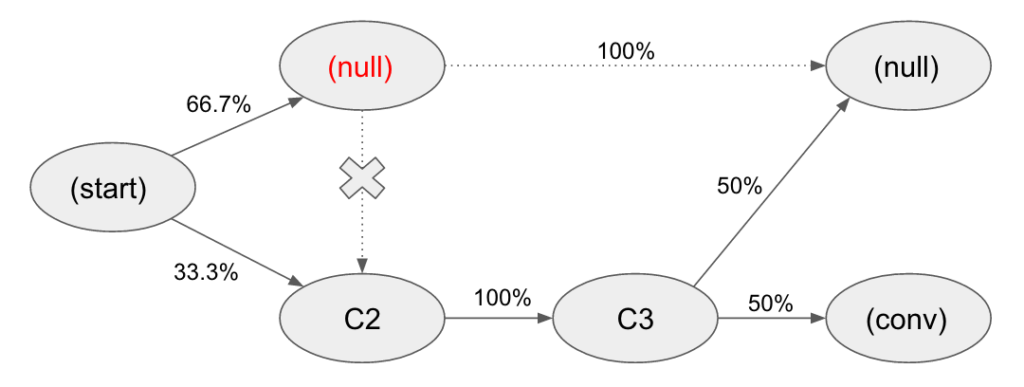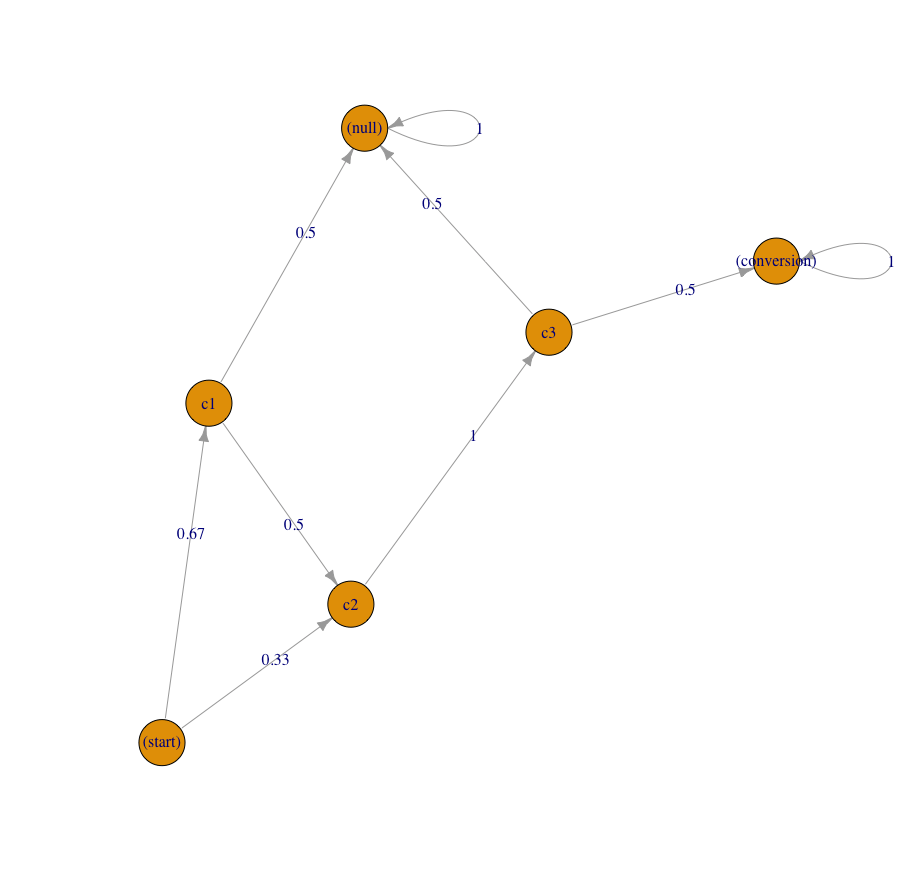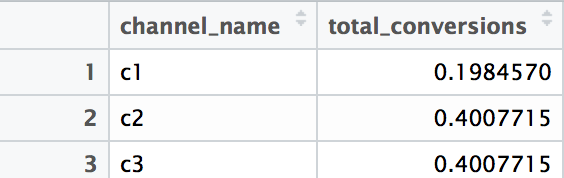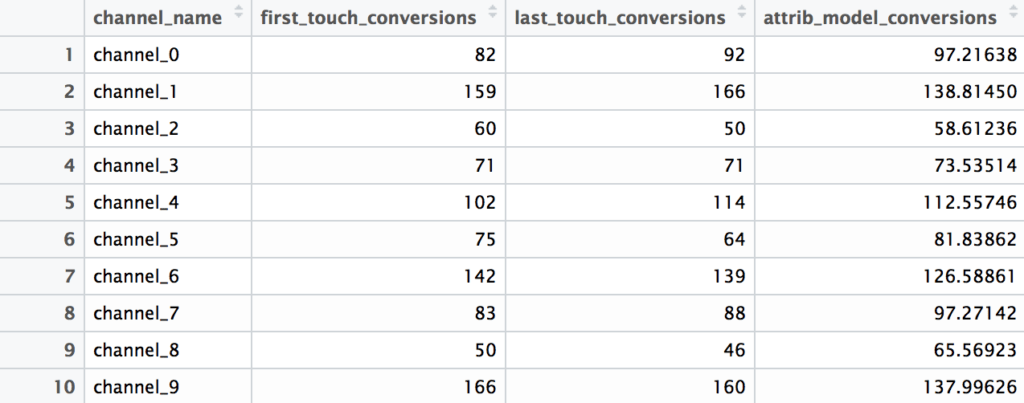[This article was first published on R language – AnalyzeCore by Sergey Bryl' – data is beautiful, data is a story, and kindly contributed to R-bloggers]. (You can report issue about the content on this page here)
Want to share your content on R-bloggers? click here if you have a blog, or here if you don't.

Using Markov chains allow us to switch from Google Analytics heuristic models to probabilistic ones. By computing the model and estimating transition probabilities we can attribute every channel/touchpoint
As we know, a customer usually goes through a path/sequence of different channels/touchpoints before a purchase in e-commerce or conversion in other areas. In Google Analytics we can find some touchpoints more likely to assist to conversion than others that more likely to be last-click touchpoint.

As most of the channels are paid for (in terms of money or time spent), it is vital to have an algorithm for distributing conversions and the value between those channels and compare with their costs instead of crediting e.g. last non-direct channel only. This is a Multi-Channel Attribution Model problem.

A definition by Google Analytics helps: an Attribution Model is a rule, or set of rules, that determines how credit for sales and conversions is assigned to touchpoints in conversion paths.

Nowadays, Google Analytics provides seven (!) predefined attribution models and even a custom model that you can adapt to your case. However, there are some aspects that I don’t like about the Google Analytics approach, which is why I started research on this area. I’m sure this is a very interesting field for analysts and marketers. I’m going to publish a sequence of posts about alternative (relatively to Google Analytics) Attribution Model concepts, some ideas for solving issues that you would face in practice when implementing them, and R code for computing them (as always).

What I don’t like about the GA approach:

• You have to make a choice or managerial decision regarding which model to use and why. You can see different results with different models but which one is more correct? In other words, GA provides heuristic models with their pros and cons,
• The data are aggregated and anonymized and you can’t mine deeper if you want,
• You can’t take into account paths without conversions but this would be interesting.

Pros of GA:

• You don’t need to organize a storage and infrastructure for collecting data,
• You are provided with a range of heuristic models,
• It is pretty easy and free to use.

Therefore, if you are relatively small company it would be logical to use the GA’s approach but if you see the results of attribution would have a significant impact on marketing budgets, product prices, understanding customer journeys, etc. or you have the necessary data collected, you can explore ideas that I’m going to share.

I focused on the Markov chains concept for attribution in this article mainly. In the second post of the series, we will study practical aspects of its implementation.

### Attribution Model based on Markov chains concept

Using Markov chains allow us to switch from heuristic models to probabilistic ones. We can represent every customer journey (sequence of channels/touchpoints) as a chain in a directed Markov graph where each vertex is a possible state (channel/touchpoint) and the edges represent the probability of transition between the states (including conversion.) By computing the model and estimating transition probabilities we can attribute every channel/touchpoint.

Let’s start with a simple example of the first-order or “memory-free” Markov graph for better understanding the concept. It is called “memory-free” because the probability of reaching one state depends only on the previous state visited.

For instance, customer journeys contain three unique channels C1, C2, and C3. In addition, we should manually add three special states to each graph: (start), (conversion) and (null). These additional states represent starting point, purchase or conversion, and unsuccessful conversion. Transitions from identical channels are possible (e.g. C1 -> C1) but can be omitted for different reasons.

Let’s assume we have three customer journeys:

C1 -> C2 -> C3 -> purchase

C1 -> unsuccessful conversion

C2 -> C3 -> unsuccessful conversion

Due to the approach, we will add extra states (see column 2 of the following table) and split for pairs (see column 3):

1 – Customer journey2 – Transformation3 – Splitting for pairs
C1 -> C2 -> C3 -> purchase(start) -> C1 -> C2 -> C3 -> (conversion)(start) -> C1, C1 -> C2, C2 -> C3, C3 -> (conversion)
C1(start) -> C1 -> (null)(start) -> C1, C1 -> (null)
C2 -> C3(start) -> C2 -> C3 -> (null)(start) -> C2, C2 -> C3, C3 -> (null)

After this, we need to calculate the probabilities of the transition from state to state:

fromtoprobabilitytotal probability
(start)C11/366.7%
(start)C11/3
(start)C21/333.3%
total from (start)3/3
C1C21/250%
C1(null)1/250%
total from C12/2
C2C31/2100%
C2C31/2
total from C22/2
C3(conversion)1/250%
C3(null)1/250%
total from C32/2

Finally, we can plot the model:The last step is to estimate every channel/touchpoint. It is pretty easy to do this by using the principle of Removal Effect.  The core of Removal Effect is to remove each channel from the graph consecutively and measure how many conversions (or how much value) could be made (earned) without the one. The logic is the following: if we obtain N conversions without a certain channel/touchpoint compared to total conversions T of the complete model, that means the channel reflects the change in total conversions (or value). After all, channels/touchpoints are estimated: we have to weight them because the total sum of (T – Ni) would be bigger than T and normally it is.

Another effective way to measure the Removal Effect is in percentages e.g. the channel affected conversion probabilities by X %.

Let’s see how this works in our simplified example. Removing a channel/touchpoint from the graph means we should replace it in channel pairs. In case the channel exists in a «from» state, we will replace it with NA (and then omit this pair) and we will replace the channel with (null) if it is in a «to» state. In other words, we will not have paths from the channel and will transit from the other channels to (null) state when the channel is in a «to» part.

Removal Effect for C1 channel looks like the following:Therefore, the probability of conversion of the complete model is 33.3% (0.667 * 0.5 * 1 * 0.5 + 0.333 * 1 * 0.5.) The probability of conversion after removing the C1 channel is 16.7% (0.333 * 1 * 0.5.) Therefore, the channel C1 removal effect is 0.5 (1 – 0.167 / 0.333.) In other words, if we didn’t have the channel C1 in customer journeys we would lose 50% of conversions.

The removal effect of both C2 and C3 is 1 because we would lose all 100% conversion (1 – 0 / 0.333).

In addition, we need to weight the indexes and multiply them by total number of conversions (1 in our case):

• C1: 0.5 / (0.5 + 1 + 1) = 0.2 * 1 conversion = 0.2
• C2: 1 / (0.5 + 1 + 1) = 0.4 * 1 conversion = 0.4
• C3: 1 / (0.5 + 1 + 1) = 0.4 * 1 conversion = 0.4

Therefore, we distributed 1 conversion for all channels.

I think the method is clear for you now. Let’s do this with R language. We will create the simplified example as above and simulate a dataset that looks like real data of customer journeys in addition.

Hint: there is a great R-package (ChannelAttribution) available on CRAN. It is really nice because of its simplicity and speed but I found some issues in the early stages of my investigation. That is why I’ve done all the work manually. Luckily, the author of the package (Davide Altomare) replied to my comments and fixed the bugs. Therefore, I’ve obtained the equal results of my manual approach and the package from the 1.8 version. Thus, please be sure that you have the latest version of the package installed.

The following is the R code for simple example that we reviewed above:

click to expand R code

```library(dplyr)
library(reshape2)
library(ggplot2)
library(ggthemes)
library(ggrepel)
library(RColorBrewer)
library(markovchain)

##### simple example #####
# creating a data sample
df1 <- data.frame(path = c('c1 > c2 > c3', 'c1', 'c2 > c3'), conv = c(1, 0, 0), conv_null = c(0, 1, 1))

# calculating the model
mod1 <- markov_model(df1,
var_path = 'path',
var_conv = 'conv',
var_null = 'conv_null',
out_more = TRUE)

# extracting the results of attribution
df_res1 <- mod1\$result

# extracting a transition matrix
df_trans1 <- mod1\$transition_matrix
df_trans1 <- dcast(df_trans1, channel_from ~ channel_to, value.var = 'transition_probability')

### plotting the Markov graph ###
df_trans <- mod1\$transition_matrix

# adding dummies in order to plot the graph
df_dummy <- data.frame(channel_from = c('(start)', '(conversion)', '(null)'),
channel_to = c('(start)', '(conversion)', '(null)'),
transition_probability = c(0, 1, 1))
df_trans <- rbind(df_trans, df_dummy)

# ordering channels
df_trans\$channel_from <- factor(df_trans\$channel_from,
levels = c('(start)', '(conversion)', '(null)', 'c1', 'c2', 'c3'))
df_trans\$channel_to <- factor(df_trans\$channel_to,
levels = c('(start)', '(conversion)', '(null)', 'c1', 'c2', 'c3'))
df_trans <- dcast(df_trans, channel_from ~ channel_to, value.var = 'transition_probability')

# creating the markovchain object
trans_matrix <- matrix(data = as.matrix(df_trans[, -1]),
nrow = nrow(df_trans[, -1]), ncol = ncol(df_trans[, -1]),
dimnames = list(c(as.character(df_trans[, 1])), c(colnames(df_trans[, -1]))))
trans_matrix[is.na(trans_matrix)] <- 0
trans_matrix1 <- new("markovchain", transitionMatrix = trans_matrix)

# plotting the graph
plot(trans_matrix1, edge.arrow.size = 0.35)
```

We have obtained the visualization of Markov graph, transition matrix (df_trans1 data frame) and the attribution results that look pretty the same with our calculations (df_res1 data frame):Let’s simulate a dataset that looks like real data of customer journeys. We assume that all paths were finished with the purchase/conversion.

click to expand R code

```# simulating the "real" data
set.seed(354)
df2 <- data.frame(client_id = sample(c(1:1000), 5000, replace = TRUE),
date = sample(c(1:32), 5000, replace = TRUE),
channel = sample(c(0:9), 5000, replace = TRUE,
prob = c(0.1, 0.15, 0.05, 0.07, 0.11, 0.07, 0.13, 0.1, 0.06, 0.16)))
df2\$date <- as.Date(df2\$date, origin = "2015-01-01")
df2\$channel <- paste0('channel_', df2\$channel)

# aggregating channels to the paths for each customer
df2 <- df2 %>%
arrange(client_id, date) %>%
group_by(client_id) %>%
summarise(path = paste(channel, collapse = ' > '),
# assume that all paths were finished with conversion
conv = 1,
conv_null = 0) %>%
ungroup()

# calculating the models (Markov and heuristics)
mod2 <- markov_model(df2,
var_path = 'path',
var_conv = 'conv',
var_null = 'conv_null',
out_more = TRUE)

# heuristic_models() function doesn't work for me, therefore I used the manual calculations
#h_mod2 <- heuristic_models(df2, var_path = 'path', var_conv = 'conv')

df_hm <- df2 %>%
mutate(channel_name_ft = sub('>.*', '', path),
channel_name_ft = sub(' ', '', channel_name_ft),
channel_name_lt = sub('.*>', '', path),
channel_name_lt = sub(' ', '', channel_name_lt))
# first-touch conversions
df_ft <- df_hm %>%
group_by(channel_name_ft) %>%
summarise(first_touch_conversions = sum(conv)) %>%
ungroup()
# last-touch conversions
df_lt <- df_hm %>%
group_by(channel_name_lt) %>%
summarise(last_touch_conversions = sum(conv)) %>%
ungroup()

h_mod2 <- merge(df_ft, df_lt, by.x = 'channel_name_ft', by.y = 'channel_name_lt')

# merging all models
all_models <- merge(h_mod2, mod2\$result, by.x = 'channel_name_ft', by.y = 'channel_name')
colnames(all_models)[c(1, 4)] <- c('channel_name', 'attrib_model_conversions')
```

The results are the following (all_models data frame):In addition, let’s create some visualizations for transition matrix and the difference between heuristic models and attribution model with the following code:

click to expand R code

```############## visualizations ##############
# transition matrix heatmap for "real" data
df_plot_trans <- mod2\$transition_matrix

cols <- c("#e7f0fa", "#c9e2f6", "#95cbee", "#0099dc", "#4ab04a", "#ffd73e", "#eec73a",
"#e29421", "#e29421", "#f05336", "#ce472e")
t <- max(df_plot_trans\$transition_probability)

ggplot(df_plot_trans, aes(y = channel_from, x = channel_to, fill = transition_probability)) +
theme_minimal() +
geom_tile(colour = "white", width = .9, height = .9) +
scale_fill_gradientn(colours = cols, limits = c(0, t),
breaks = seq(0, t, by = t/4),
labels = c("0", round(t/4*1, 2), round(t/4*2, 2), round(t/4*3, 2), round(t/4*4, 2)),
guide = guide_colourbar(ticks = T, nbin = 50, barheight = .5, label = T, barwidth = 10)) +
geom_text(aes(label = round(transition_probability, 2)), fontface = "bold", size = 4) +
theme(legend.position = 'bottom',
legend.direction = "horizontal",
panel.grid.major = element_blank(),
panel.grid.minor = element_blank(),
plot.title = element_text(size = 20, face = "bold", vjust = 2, color = 'black', lineheight = 0.8),
axis.title.x = element_text(size = 24, face = "bold"),
axis.title.y = element_text(size = 24, face = "bold"),
axis.text.y = element_text(size = 8, face = "bold", color = 'black'),
axis.text.x = element_text(size = 8, angle = 90, hjust = 0.5, vjust = 0.5, face = "plain")) +
ggtitle("Transition matrix heatmap")

# models comparison
all_mod_plot <- melt(all_models, id.vars = 'channel_name', variable.name = 'conv_type')
all_mod_plot\$value <- round(all_mod_plot\$value)
# slope chart
pal <- colorRampPalette(brewer.pal(10, "Set1"))
ggplot(all_mod_plot, aes(x = conv_type, y = value, group = channel_name)) +
theme_solarized(base_size = 18, base_family = "", light = TRUE) +
scale_color_manual(values = pal(10)) +
scale_fill_manual(values = pal(10)) +
geom_line(aes(color = channel_name), size = 2.5, alpha = 0.8) +
geom_point(aes(color = channel_name), size = 5) +
geom_label_repel(aes(label = paste0(channel_name, ': ', value), fill = factor(channel_name)),
alpha = 0.7,
fontface = 'bold', color = 'white', size = 5,
box.padding = unit(0.25, 'lines'), point.padding = unit(0.5, 'lines'),
max.iter = 100) +
theme(legend.position = 'none',
legend.title = element_text(size = 16, color = 'black'),
legend.text = element_text(size = 16, vjust = 2, color = 'black'),
plot.title = element_text(size = 20, face = "bold", vjust = 2, color = 'black', lineheight = 0.8),
axis.title.x = element_text(size = 24, face = "bold"),
axis.title.y = element_text(size = 16, face = "bold"),
axis.text.x = element_text(size = 16, face = "bold", color = 'black'),
axis.text.y = element_blank(),
axis.ticks.x = element_blank(),
axis.ticks.y = element_blank(),
panel.border = element_blank(),
panel.grid.major = element_line(colour = "grey", linetype = "dotted"),
panel.grid.minor = element_blank(),
strip.text = element_text(size = 16, hjust = 0.5, vjust = 0.5, face = "bold", color = 'black'),
strip.background = element_rect(fill = "#f0b35f")) +
labs(x = 'Model', y = 'Conversions') +
ggtitle('Models comparison') +
guides(colour = guide_legend(override.aes = list(size = 4)))
```

We obtained the heatmap of the transition matrix:And models comparison indicates substantial differences to existing heuristics such as «first-click» and «last-click» as well as alternative attribution approach:In the next post, we will study how to do attribution based on the first-order Markov chains in practice. We will see that while the method is pretty simple, there will be a lot of questions that we need to answer and, therefore, apply to the R script. Specifically, we will study how to:

• define the retrospective period for analysis,
• manage several purchases per reporting period,
• deal with paths without purchase,
• replace «direct» (or any other unknown) channel with a non-direct or known one,
• calculate both conversions and revenue,
• etc.

Therefore, the next post will be practical oriented, don’t miss itP.S.: just found that Lunametrics also has published the post about.

SaveSave

SaveSave

SaveSave

To leave a comment for the author, please follow the link and comment on their blog: R language – AnalyzeCore by Sergey Bryl' – data is beautiful, data is a story.

R-bloggers.com offers daily e-mail updates about R news and tutorials about learning R and many other topics. Click here if you're looking to post or find an R/data-science job.
Want to share your content on R-bloggers? click here if you have a blog, or here if you don't.

# Never miss an update! Subscribe to R-bloggers to receive e-mails with the latest R posts.(You will not see this message again.)

Click here to close (This popup will not appear again)